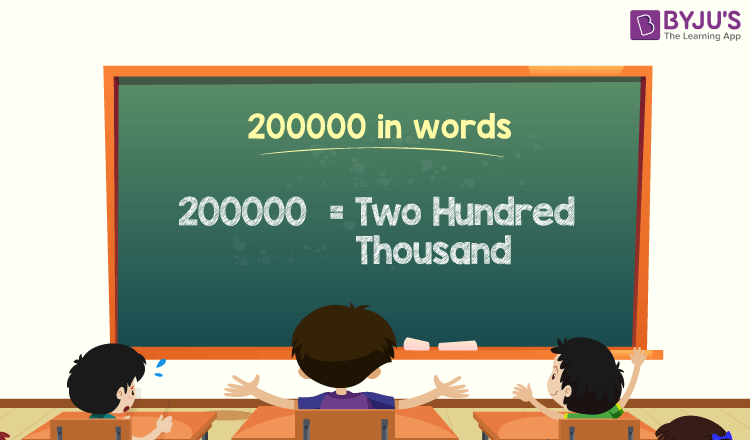# 200000 in Words

200000 in words is Two Hundred Thousand. For example, if you spent Rs. 200000 in five months, then you can write, “I have spent Rs. Two Hundred Thousand in five months”. The number name of any number can be written using the place value of the number such as ones, tens, hundreds, thousands, and so on.

 200000 in Words: Two Hundred Thousand

In this article, let us discuss how to write 200000 in words, and look at the solved examples in detail.

## How to Write 200000 in Words?To write the number name of 200000, first, identify the place value of each digit of the given number 200000. Hence, for the number 200000,

1’s place of 200000 is 0

10’s place of 200000 is 0.

100’s place of 200000 is 0.

1000’s place of 200000 is 200.

The place value chart for the number 200000 is as follow:

 Thousands Hundreds Tens Ones 200 0 0 0

Therefore, the number 200000 in words is Two Hundred Thousand.

### Examples

Example 1:

Find the value of 150000 + 50000. Describe the value in words.

Solution:

Given expression: 150000 + 50000

⇒ 150000 + 50000 = 200000

So, the value of 150000 + 50000 is 200000.

Hence, 200000 in words is two hundred thousand.

Example 2:

Express the value of two hundred thousand minus thirty thousand in words.

Solution:

Two hundred thousand = 200000

Thirty thousand = 30000

Two hundred thousand minus thirty thousand = 200000 – 30000 = 170000

Hence, the value of two hundred thousand minus thirty thousand is one hundred seventy thousand in words.

## Frequently Asked Questions on 200000 in Words

Q1

### Write 200000 in words.

200000 in words is two hundred thousand.

Q2

### Express the value 100000 + 100000 in words.

Simplifying 100000 + 100000, we get 200000. Hence, 200000 in words is two hundred thousand.

Q3

### How to write two hundred thousand in numbers?

Two hundred thousand in numbers is 200000.

Q4

### Is the number 200000 an even number?

Yes, the number 200000 is an even number.

Q5

### Is 200000 a prime number?

No, 200000 is not a prime number.